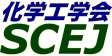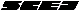## \$B9V1i%W%m%0%i%`!J2q>l!&F|DxJL!K(B

### M\$B2q>l(B

\$B:G=*99?7F|;~!'(B2012-08-20 15:02:17

\$BBh(B1\$BF|(B
\$B9V1i(B
\$B;~9o(B
\$B9V1i(B
\$BHV9f(B
\$B9V1iBjL\!?H/I=\$B%-!<%o!<%I(B\$BJ,N`(B
\$BHV9f(B
\$B\$BHV9f(B
\$B%7%s%]%8%&%`(B \$B!c%(%M%k%.!<%7%s%]%8%&%`!d(B
(9:20\$B!A(B10:40)\$B!!(B(\$B:BD9(B \$B>.AR(B \$BM5D>(B)
9:20\$B!A(B 9:40M102CaCl2\$B!?%7%e%&;@%"%k%^%\$%HJ#9g:`\$ND4@=\$H\$=\$N?e>x5\$<}CeFC@-(B
(\$B6bBtBg1!<+(B) \$B!{(B(\$B3X(B)\$B?[K,(B \$BM4;J(B \$B!&(B \$B>.?9(B \$B9-L@(B \$B!&(B (\$B6bBtBgM}9)(B) (\$B@5(B)\$B5bED(B \$B44IW(B
Calcium chloride
Anodized alumina
Water vapor sorption
S-2422
9:40\$B!A(B 10:00M103\$BDc29GSG.3hMQ\$N\$?\$a\$NL55!?eOBJ*7O9bC_G.L)EY:`NA\$K4X\$9\$k8&5f(B
(\$BL>Bg1!9)(B) \$B!{(B(\$B3X(B)\$B30 \$B!&(B (\$B@5(B)\$B7&ED(B \$B8w9((B \$B!&(B (\$B@5(B)\$B>>ED(B \$B?N
Chemical heat pump
Hydration
S-2650
10:00\$B!A(B 10:20M104\$BJ#9g?e;@2=J*7O2=3XC_G.:`\$N7+JV\$7H?1~BQ5W@-(B
(\$BEl9)Bg(B) \$B!{(B(\$B@5(B)\$BN-(B \$B=f0l(B \$B!&(B \$B:4F#(B \$B5ACR(B \$B!&(B \$B?eLn(B \$B=T;K(B \$B!&(B (\$B3X(B)\$B@PHt(B \$B9(OB(B \$B!&(B (\$B@5(B)\$B2CF#(B \$BG75.(B
Chemical Heat Storage
Gas Solid Reaction
Mixed Hydroxide
S-245
10:20\$B!A(B 10:40M105\$B4uEZN`J#9g?e;@2=J*\$NC&?eH?1~G.\$NB,Dj(B
(\$BEl9)Bg1!M}9)(B) \$B!{(B(\$B3X(B)\$B@PHt(B \$B9(OB(B \$B!&(B (\$BEl9)Bg86;RO'8&(B) (\$B@5(B)\$BN-(B \$B=f0l(B \$B!&(B (\$B@5(B)\$B2CF#(B \$BG75.(B
Chemical heat pump
Rare earth mixed hydroxide
Differential scanning calorimetry
S-2437
(10:40\$B!A(B12:00)\$B!!(B(\$B:BD9(B \$B5bED(B \$B44IW(B)
10:40\$B!A(B 11:00M106\$B%1%_%+%k%R!<%H%]%s%WH?1~:`86NA\$H\$7\$F\$N@P9Q\$N;:COJLFC@-(B
(\$B@iMUBg1!(B) \$B!{(B(\$B3X(B)\$BM{(B \$B=St&(B \$B!&(B (\$B@5(B)\$B>.AR(B \$BM5D>(B
Chemical Heat Pump
Calcium Sulfate
Reactivity
S-2640
11:00\$B!A(B 11:20M107\$BKDD%2=%0%i%U%!%\$%H\$rMQ\$\$\$?;@2=%+%k%7%&%`(B/\$B?e7O%1%_%+%k%R!<%H%]%s%W\$N3+H/(B
(\$BEl9)Bg1!M}9)(B) \$B!{(B(\$B3X(B)Kariya Jun \$B!&(B (\$B3X(B)Kim Seon Tae \$B!&(B (\$BEl9)Bg86;RO'8&(B) (\$B@5(B)Ryu Junichi \$B!&(B (\$B@5(B)Kato Yukitaka
heat pump
calcium oxide
graphite
S-2137
11:20\$B!A(B 11:40M108The effects of density on Mg(OH)2 - expanded graphite pellets for a chemical heat pump
(\$BEl9)Bg(B) \$B!{(B(\$B3X(B)Zamengo Massimiliano \$B!&(B (\$B3X(B)Kim Seon Tae \$B!&(B (\$B@5(B)Ryu Junichi \$B!&(B (\$B@5(B)Kato Yukitaka
chemical heat pump
expanded graphite
heat storage
S-2461
11:40\$B!A(B 12:00M109Na2S\$B?eOBH?1~MxMQ%1%_%+%kC_G.\$NJ#9g:`NA\$N3+H/(B
(\$B \$B!&(B \$B>.;3(B \$BM:Li(B \$B!&(B \$BLn8}(B \$BB@O:(B \$B!&(B \$BA}Eg(B \$B@69'(B \$B!&(B \$B4X:,(B \$BM42p(B
Chemical energy storage
Sodium sulphide hydrate
Thermal energy storage
S-2471
(13:00\$B!A(B13:40)\$B!!(B(\$B;J2q(B \$BCf3@(B \$BN4M:(B)
13:00\$B!A(B 13:40M113[\$B0MMj9V1i(B]\$BDcC:AG
(\$BElBg@88&(B) \$B!{4dA%(B \$BM3H~;R(B
HEMS
Demand Response
S-2541
(13:40\$B!A(B15:20)\$B!!(B(\$B:BD9(B \$B7&ED(B \$B8w9((B)
13:40\$B!A(B 14:00M115(\$B9V1iCf;_(B)

100216
14:00\$B!A(B 14:20M116\$B9b29O'G.\$rMxMQ\$7\$?G.2=3X:F@8\$N9b=cEY%"%k%_%J4I\$K\$h\$k%a%?%s?e>x5\$2~
(\$BAaBg1!(B) \$B!{(B(\$B3X(B)\$B2CF#(B \$BBs??(B \$B!&(B (\$BAaBg(B) (\$B@5(B)\$BCf3@(B \$BN4M:(B \$B!&(B (\$BAaBg1!(B) \$B=EED(B \$BM:N<(B \$B!&(B (\$BF|K\HD>K;R(B) \$BED8}(B \$B2mJ8(B \$B!&(B \$BD9Eh(B \$BNw?N(B
steam reforming
chemical requperation
high temperature
S-2304
14:20\$B!A(B 14:40M117\$BB?9&eNP2=\$K\$h\$k%R!<%H%"%\$%i%s%I4KOB8z2L(B
(\$B6bBtBg1!(B) \$B!{(B(\$B3X(B)Manirakiza Eric \$B!&(B (\$B>.>>@:N}(B) \$B1|C+(B \$B989((B \$B!&(B (\$B6bBtBgM}9)(B) (\$B@5(B)\$B5bED(B \$B44IW(B \$B!&(B (\$B@5(B)\$B@%8M(B \$B>OJ8(B \$B!&(B (\$B@5(B)\$BBgC+(B \$B5H@8(B
Porous Ceramic
Uraban Heat Island
Heat and mass transfer
S-2467
14:40\$B!A(B 15:00M118\$BH/EE%3%9%H\$HEENO6!5k\$K4X\$9\$k0l9M;!(B
(\$B@EBg9)(B) \$B!{(B(\$B@5(B)\$B>>ED(B \$BCR(B \$B!&(B \$B;T66(B \$BNI??(B
cost of power generation
nuclear power
coal-fired plant
S-2468
15:00\$B!A(B 15:20M119\$B%a%+%N%1%_%+%kK!\$H2CG.K!\$rMxMQ\$7\$?2
(\$BElKLBg1!4D6-(B) \$B!{(B(\$B3X(B)\$B0KF#(B \$B;KO/(B \$B!&(B (\$BElKLBgB?858&(B) (\$B@5(B)\$Bc7F#(B \$BJ8NI(B \$B!&(B (\$B@5(B)\$BD%(B \$BB6Ip(B \$B!&(B (\$BK-EDCf8&(B) \$B>>K\(B \$BK~(B
mechanochemistry
sludge
hydrogen
S-2482
(15:20\$B!A(B16:00)\$B!!(B(\$B;J2q(B \$BEDCf(B \$B9LB@O:(B)
15:20\$B!A(B 16:00M120[\$BE8K>9V1i(B]\$B9b5!G=%<%*%i%\$%H(BAQSOA\$B\$N3+H/\$*\$h\$S
(\$B;0I)
Desiccant
Zeolite
S-2133
(16:00\$B!A(B17:00)\$B!!(B(\$B:BD9(B \$BN-(B \$B=f0l(B)
16:00\$B!A(B 16:20M122\$B%>%k(B-\$B%2%kK!\$HEE5\$1KF0K!\$rJ;MQ\$9\$k%7%j%+!?%"%k%_%K%&%`J#9g5[Ce:`\$ND4@=(B
(\$B6bBtBg1!<+(B) \$B!{(B(\$B3X(B)\$B>>0f(B \$B9/0l(B \$B!&(B (\$B3X(B)\$B?[K,(B \$BM4;J(B \$B!&(B (\$B6bBtBgM}9)(B) (\$B@5(B)\$B5bED(B \$B44IW(B
sol-gel method
electrophoretic deposition
silica/aluminum composite
S-2719
16:20\$B!A(B 16:40M123\$BD>@\@\?(<0G.8r49K!\$rMQ\$\$\$?@xG.C_G.AuCV\$N@-G=FC@-(B
(\$BKLBg(B) \$B!{(B(\$B3X(B)\$BG=B<(B \$B5.9((B \$B!&(B \$BDZED(B \$B2m8y(B \$B!&(B \$B2-Cf(B \$B7{G7(B \$B!&(B (\$B@5(B)\$B=);3(B \$BM'9((B
Phase Change Material (PCM)
Latent heat storage
Thermal energy storage
S-2830
16:40\$B!A(B 17:00M124\$B29EYMzNrK!\$K\$h\$k@xG.C_G.:`\$N@-G=FC@-\$NI>2A(B
(\$BEl5~EE5!Bg1!M}9)(B) \$B!{(B(\$B3X(B)\$BEhB<(B \$B7CN\$(B \$B!&(B (\$BEl5~EE5!BgM}9)(B) (\$B@5(B)\$BLZB<(B \$BFs;0IW(B \$B!&(B (\$BEl5~EE5!Bg1!M}9)(B) (\$B@5(B)\$B>.@n(B \$B1Q@8(B
thermal energy storage
phase change material
T-history method
S-2674

\$BBh(B2\$BF|(B
\$B9V1i(B
\$B;~9o(B
\$B9V1i(B
\$BHV9f(B
\$B9V1iBjL\!?H/I=\$B%-!<%o!<%I(B\$BJ,N`(B
\$BHV9f(B
\$B\$BHV9f(B
\$B%7%s%]%8%&%`(B \$B!c%(%M%k%.!<%7%s%]%8%&%`!d(B
(9:00\$B!A(B10:00)\$B!!(B(\$B:BD9(B \$B?@86(B \$B?.;V(B)
9:00\$B!A(B 9:20M201\$BFqJ,2r@-M-5!J*\$NG.2=3XE*%,%92=FC@-\$N8!F\$(B
(\$BAO2ABg9)(B) \$B!{(B(\$B3X(B)\$BCfEg(B \$B9d;K(B \$B!&(B \$B>>K\(B \$B@6?M(B \$B!&(B (\$B@5(B)\$BEOIt(B \$BAO;N(B \$B!&(B (\$B@5(B)\$B0fED(B \$B=\0l(B \$B!&(B (\$B@5(B)\$B>>;3(B \$BC#(B \$B!&(B (\$B@5(B)\$B;3K\(B \$B1QIW(B
Methane fermentation residue
Waterweed
Gasification
S-2716
9:20\$B!A(B 9:40M202H2\$B\$*\$h\$S(BCO\$B@8@.\$K5Z\$\\$9(BO2/CO2\$B%,%92=\$NFC0[@-(B
(\$BEl9)Bg(B) \$B!{(B(\$B@5(B)\$BEOIt(B \$B90C#(B \$B!&(B Kiatpanachart Dejudom \$B!&(B \$B9S0f(B \$B0jLi(B \$B!&(B (\$B@5(B)\$B2,:j(B \$B7r(B
O2/CO2 coal gasification
detailed chemical reaction
S-2957
9:40\$B!A(B 10:00M203Characteristics of volatile reactions and char gasification in O2/CO2 coal combustion
(Tokyo Inst. Tech.) \$B!{(BLi Xiaofan \$B!&(B Arai Fumiya \$B!&(B Kiatpanachart Dejudom \$B!&(B (\$B@5(B)Watanabe Hirotatsu \$B!&(B (\$B@5(B)Okazaki Ken
O2/CO2 combustion
coal
CO2 reactivity
S-2968
(10:00\$B!A(B11:00)\$B!!(B(\$B:BD9(B \$BLnED(B \$BNh<#(B)
10:00\$B!A(B 10:20M204\$B3lC:MxMQ2PNOH/EE%W%i%s%H\$NG.8zN(8~>eK!\$N8!F\$(B
(\$B4tIlBg1!(B) \$B!{(B(\$B@5(B)\$B?@86(B \$B?.;V(B \$B!&(B (\$B3X(B)\$BIp;3(B \$B>49((B \$B!&(B (\$B@5(B)\$B7(It(B \$BOB90(B \$B!&(B (\$B@5(B)\$B
Low rank coal
Power plant
Thermal efficiency
S-2113
10:20\$B!A(B 10:40M205\$BDcIJ0LC:AG;q8;\$N2~
(\$B5~Bg9)(B) \$B!{(B(\$B3X(B)\$BF#J/(B \$BBgM5(B \$B!&(B (\$B@5(B)\$BiCED(B \$BN40l(B \$B!&(B (\$B@5(B)\$B;01:(B \$B9'0l(B
self-ignition tendency
S-2802
10:40\$B!A(B 11:00M206\$BLZ
(\$B2,;3Bg1!4D6-@8L?(B) \$B!{(B(\$B3X(B)\$BEOJU(B \$BBgCR(B \$B!&(B (\$B@5(B)Uddin Md. Azhar \$B!&(B (\$B@5(B)\$B2CF#(B \$B2E1Q(B
Woody Biomass
Biomass Tar
Catalytic Decomposition
S-2179
(11:00\$B!A(B12:00)\$B!!(B(\$B:BD9(B \$BEOIt(B \$B90C#(B)
11:00\$B!A(B 11:20M207\$B%]%s%]%s>uE44p?(G^\$rMQ\$\$\$?%P%\$%*%^%9M3Mh%?!<%k\$N2~
(\$B90A0BgM}9)(B) \$B!{(B(\$B3X(B)Kaewpanha Malinee \$B!&(B (\$B90A0Bg?7%(%M!<8&(B) (\$B@5(B)\$B41(B \$B9q@6(B \$B!&(B \$B8b(B \$B2BIK(B \$B!&(B (\$B@D?98)9)Am8&(B) (\$B6&(B)\$B3k@>(B \$BM5(B \$B!&(B (\$B90A0Bg?7%(%M!<8&(B) (\$B@5(B)\$B0\$I[(B \$BN\$Ds(B
Biomass tar
Steam reforming
Pompom-like catalyst
S-2112
11:20\$B!A(B 11:40M208\$B%P%\$%*%^%9%?!<%kDc292~
(\$B72Bg(B) \$B!{(B(\$B@5(B)Sirimirin Meesuk \$B!&(B \$B:4F#(B \$B@1EM(B \$B!&(B \$B>>Eh(B \$B:L(B \$B!&(B (\$B@5(B)\$B:4F#(B \$BOB9%(B \$B!&(B (\$B@5(B)\$BJuED(B \$B63G7(B
\$B8GBN?(G^(B
\$B%P%\$%*%^%9(B
\$BDc29%,%92=(B
S-2326
11:40\$B!A(B 12:00M2093\$B<
(\$B72Bg1!9)(B) \$B!{(B(\$B3X(B)\$BD.ED(B \$BM&Lo(B \$B!&(B (\$B72Bg9)(B) \$B=U;3(B \$B9IJ?(B \$B!&(B (\$B72Bg1!9)(B) (\$B@5(B)\$BLnED(B \$BNh<#(B
biomass
bio-fuel
fluidized bed
S-2363
(13:00\$B!A(B14:00)\$B!!(B(\$B:BD9(B \$B5A2H(B \$BN<(B)
13:00\$B!A(B 13:20M213(\$B9V1iCf;_(B)

10036
13:20\$B!A(B 13:40M214\$B3lC:%A%c!x5\$%,%92=\$NB.EYO@\$KBP\$9\$kG.J,2r@-41G=4p\$N1F6A(B
(\$B6eBgC:AG%;(B) \$B!{(B(\$B@5(B)\$B9)F#(B \$B??Fs(B \$B!&(B (\$B6eBgAmM}9)(B) \$BDEF`LZ(B \$B>J8c(B \$B!&(B (\$B6eBg@hF38&(B) (\$B@5(B)\$BB'1J(B \$B9TMG(B \$B!&(B (\$B@5(B)\$BNS(B \$B=a0lO:(B
\$B@PC:(B
\$B%,%92=(B
\$BG.J,2r(B
S-2516
13:40\$B!A(B 14:00M215\$B3lC:!?%P%\$%*%^%9%A%c!<\$NL5?(G^?e>x5\$%,%92=5!9=(B
(\$B6eBg@hF38&(B) \$BH,;3(B \$BLwBe(B \$B!&(B (\$B6eBgC:AG%;(B) \$B!{(B(\$B@5(B)\$B9)F#(B \$B??Fs(B \$B!&(B (\$B6eBg@hF38&(B) (\$B@5(B)\$BB'1J(B \$B9TMG(B \$B!&(B (\$B@5(B)\$BNS(B \$B=a0lO:(B
\$B@PC:(B
\$B%,%92=(B
\$BB.EY<0(B
S-2518
(14:00\$B!A(B15:00)\$B!!(B(\$B:BD9(B \$BB'1J(B \$B9TMG(B)
14:00\$B!A(B 14:20M216\$BD>@\DLEE>.7?H?1~AuCV\$rMQ\$\$\$?9b299b05>r7o\$G\$N@PC:%,%92=H?1~B.EYB,Dj(B
(\$B5~Bg9)(B) \$B!{(B(\$B3X(B)\$B:#0f(B \$B=SJe(B \$B!&(B (\$B@5(B)\$B:{2,(B \$B1Q;J(B \$B!&(B (\$B@5(B)\$BiCED(B \$BN40l(B \$B!&(B (\$B@5(B)\$B;01:(B \$B9'0l(B
coal gasification
directly-heated reactor
Langmuir-Hinshelwood type equation
S-2796
14:20\$B!A(B 14:40M217Air gasification of biomass pellets in the auto-thermal packed bed reactor
(\$BL>Bg1!9)(B) \$B!{(B(\$B3X(B)Khedu Joseph \$B!&(B (\$BL>Bg%(%3(B) \$BI[L\(B \$BM[;R(B \$B!&(B (\$B@5(B)\$B?"LZ(B \$BJ]><(B \$B!&(B (\$BL>Bg1!9)(B) (\$B@5(B)\$B5A2H(B \$BN<(B \$B!&(B (\$BL>Bg%(%3(B) (\$B@5(B)\$B@.@%(B \$B0lO:(B
biomass
gasification
auto-thermal
S-2155
14:40\$B!A(B 15:00M218High Temperature Gasification of Plastic Waste
(\$BL>Bg1!9)(B) \$B!{(B(\$B3X(B)Nedjalkov Ivan Jordanov \$B!&(B (\$B@5(B)\$B5A2H(B \$BN<(B \$B!&(B (\$BL>Bg%(%3%H%T%"(B) (\$B@5(B)\$B?"LZ(B \$BJ]><(B \$B!&(B (\$B@5(B)\$B@.@%(B \$B0lO:(B \$B!&(B (\$B@5(B)\$BI[L\(B \$BM[;R(B
gasification
plastic
tar
S-2533

\$BBh(B3\$BF|(B
\$B9V1i(B
\$B;~9o(B
\$B9V1i(B
\$BHV9f(B
\$B9V1iBjL\!?H/I=\$B%-!<%o!<%I(B\$BJ,N`(B
\$BHV9f(B
\$B\$BHV9f(B
\$B%7%s%]%8%&%`(B \$B!c%(%M%k%.!<%7%s%]%8%&%`!d(B
(9:00\$B!A(B10:00)\$B!!(B(\$B:BD9(B \$BARK\(B \$B9@;J(B)
9:00\$B!A(B 9:20M301\$BGQ4~J*G3>F>l\$K\$*\$1\$kEAG.4I\$X\$N3%IUCe5!9=\$N2rL@(B
(\$BL>Bg1!9)(B) \$B!{(B(\$B3X(B)\$B;3K\(B \$B5.J8(B \$B!&(B (\$BL>Bg%(%3%H%T%"(B) \$BI[L\(B \$BM[;R(B \$B!&(B (\$B@5(B)\$B?"LZ(B \$BJ]><(B \$B!&(B (\$BL>Bg1!9)(B) (\$B@5(B)\$B5A2H(B \$BN<(B \$B!&(B (\$BL>Bg%(%3%H%T%"(B) (\$B@5(B)\$B@.@%(B \$B0lO:(B
waste
combustion
ash deposition
S-2300
(\$BCfItBg(B) (\$B3X(B)Jiao Facun \$B!&(B Song Wenjia \$B!&(B (\$B3X(B)Meng Ying \$B!&(B (\$B@5(B)\$B;3ED(B \$BD>?C(B \$B!&(B (\$B@5(B)\$B:4F#(B \$B8|(B \$B!&(B \$B!{(B(\$B@5(B)\$BFs5\(B \$BA1I'(B
Heavy metal vapors
toxicity of deposit
Heterogeneous nucleation
S-2325
9:40\$B!A(B 10:00M303Effect of net-modifier on the microstructure and leaching behavior of slag during high temperature gasification
(\$BCfItBg(B) \$B!{(B(\$B3X(B)\$Br2(B \$B2mU/(B \$B!&(B (\$B@5(B)\$B;3ED(B \$BD>?C(B \$B!&(B (\$B@5(B)\$B:4F#(B \$B8|(B \$B!&(B (\$B@5(B)\$BFs5\(B \$BA1I'(B
slag structure
microstructure
slag stability
S-2438
(10:00\$B!A(B11:00)\$B!!(B(\$B:BD9(B \$BFs5\(B \$BA1I'(B)
10:00\$B!A(B 10:20M304\$B@PC:%,%92=%,%9\$rG3NA\$H\$7\$FMQ\$\$\$?>l9g\$N(BSOFC\$BG3NA6K\$K\$*\$1\$k=tNt2=H?1~\$K4X\$9\$k8&5f(B
(\$B;:Am8&(B) \$B!{(B(\$B@5(B)Kuramoto Koji \$B!&(B (\$B@5(B)Fukushima Toshiyo \$B!&(B (\$B@5(B)Matsuoka Koichi \$B!&(B (\$B@5(B)Suzuki Yoshizo \$B!&(B (\$BL>Bg(B) (\$B@5(B)Yoshiie Ryo \$B!&(B (\$B@5(B)Naruse Ichiro \$B!&(B (\$B4tIlBg(B) (\$B@5(B)Kumabe Kazuhiro \$B!&(B (\$B@5(B)Moritomi Hiroshi
Solid oxide fule cell
coal gasification
trace contaminants
S-2710
10:20\$B!A(B 10:40M305CO2\$B2s<}7?9b8zN((BIGCC\$BMQ4%<0C&N2%W%m%;%9\$K\$*\$1\$kC:AG@O=P1F6A\$HBP:v(B
(\$BEECf8&(B) \$B!{(B(\$B@5(B)\$B>.NS(B \$B@?(B \$B!&(B (\$B@5(B)\$BI[@n(B \$B?.(B \$B!&(B \$BCfHx(B \$B5H?-(B
high temperature sulfur removal
carbon deposition
IGCC with CO2 capture
S-21
10:40\$B!A(B 11:00M306Carbon deposition border in the desulfurization process in IGCC
(\$BJ!2,=wBg(B) \$B!{(BYu Yu \$B!&(B (\$B@5(B)Yuichi Fujioka \$B!&(B (\$BEECf8&(B) (\$B@5(B)Makoto Kobayashi
lCarbon deposition
high temperature sulfur removal
chemical equilibrium
S-2704
(11:00\$B!A(B12:00)\$B!!(B(\$B:BD9(B \$B>.NS(B \$B@?(B)
11:00\$B!A(B 11:20M307\$BE47O5[Ce:^\$rMQ\$\$\$?4%<0C&N2%7%9%F%`\$NC&N2@-G=8!F\$(B
(\$B6eBg9)(B) \$B!{(B(\$B3X(B)\$B5\:j(B \$BM:2p(B \$B!&(B (\$B6eBg1!9)(B) (\$B@5(B)\$B>>7((B \$BMN2p(B \$B!&(B (\$B@5(B)\$BJw85(B \$B2m
IGCC
desulfuration
S-2440
11:20\$B!A(B 11:40M308\$B3h@-%3!<%/%9\$K\$h\$k(BH2S\$B5Z\$S(BCOS\$B5[CeFC@-(B
(\$B4tIlBg9)(B) \$B!{(B(\$B3X(B)\$BJ?0f(B \$B7rM'(B \$B!&(B (\$BL>Bg9)(B) (\$B3X(B)\$BDi(B \$BM:0l(B \$B!&(B (\$B@5(B)\$B>.NS(B \$B?.2p(B \$B!&(B (\$B4tIlBg9)(B) (\$B@5(B)\$BHDC+(B \$B5A5*(B
dry desulfurization
COS conversion
activated cokes
S-2634
11:40\$B!A(B 12:00M309\$B3h@-%3!<%/%9\$r5[Ce:^\$H\$7\$FMQ\$\$\$?4%<0C&N2%W%m%;%9\$K\$*\$1\$k6&B8%,%9\$N1F6A(B
(\$BL>Bg9)(B) \$B!{(B(\$B3X(B)\$BDi(B \$BM:0l(B \$B!&(B (\$B@5(B)\$B>.NS(B \$B?.2p(B \$B!&(B (\$B@5(B)\$BEDn4(B \$BLwGn(B \$B!&(B (\$B@5(B)\$BHDC+(B \$B5A5*(B
gasification
sulfuric compound
S-2397
(13:00\$B!A(B14:00)\$B!!(B(\$B:BD9(B \$B>.NS(B \$B?.2p(B)
13:00\$B!A(B 13:20M313172nmVUV\$B\$rMQ\$\$\$?(BSNCR\$BK!\$N3+H/(B
(\$B4tIlBg1!(B) \$B!{(B(\$B3X(B)\$B6aF#(B \$B8w9@(B \$B!&(B (\$B3X(B)\$BIp;3(B \$B>49((B \$B!&(B (\$B@5(B)\$B?@86(B \$B?.;V(B \$B!&(B (\$B%&%7%*EE5!(B) (\$B6&(B)\$BI)>B(B \$B@k@'(B \$B!&(B (\$B%"%/%H%j!<(B) (\$B6&(B)\$BA}0f(B \$B2j(B \$B!&(B (\$B4tIlBg1!(B) (\$B@5(B)\$B7(It(B \$BOB90(B \$B!&(B (\$B@5(B)\$B
VUV
SNCR
NOx
S-2114
13:20\$B!A(B 13:40M314\$BBg5\$05%W%i%:%^\$rMQ\$\$\$?(BSNCR\$BK!\$N3+H/(B
(\$B4tIlBg1!(B) \$B!{(B(\$B3X(B)\$BD9Fl(B \$B8w(B \$B!&(B (\$B3X(B)\$BIp;3(B \$B>49((B \$B!&(B (\$B@5(B)\$B?@86(B \$B?.;V(B \$B!&(B (\$B_7F#EE5!(B) (\$B6&(B)\$B;01:(B \$BM'5,(B \$B!&(B (\$B%"%/%H%j!<(B) (\$B6&(B)\$BA}0f(B \$B2j(B \$B!&(B (\$B4tIlBg1!(B) (\$B@5(B)\$B7(It(B \$BOB90(B \$B!&(B (\$B@5(B)\$B
Atmospheric plasma
SNCR
NOx
S-2115
13:40\$B!A(B 14:00M315\$B@PL}%3!<%/%9\$N(BKOH\$BIj3h\$GF@\$i\$l\$k3h@-C:\$N:Y9&!&I=LL2=3XFC@-(B
(\$BL>Bg1!9)(B) \$B!{(B(\$B3X(B)\$BCfEg(B \$B424p(B \$B!&(B (\$B@5(B)\$B7&ED(B \$B8w9((B \$B!&(B (\$B@5(B)\$B>>ED(B \$B?N
petroleum coke
activated carbon
S-2429
(14:00\$B!A(B15:00)\$B!!(B(\$B:BD9(B \$BHDC+(B \$B5A5*(B)
14:00\$B!A(B 14:20M316\$BMO:^Cj=P%U%i%/%7%g%M!<%7%g%sK!\$K\$h\$k@PC:!&G47k:`\$N%3!<%/%92=5sF0M=B,\$N;n\$_(B
(\$B5~Bg9)(B) \$B!{(B(\$B@5(B)\$BiCED(B \$BN40l(B \$B!&(B (\$B3X(B)\$B9bEg(B \$B7r?M(B \$B!&(B (\$B@5(B)\$B;01:(B \$B9'0l(B \$B!&(B (\$B?@8M@=9](B) \$B1|;3(B \$B7{9,(B \$B!&(B \$B_@8}(B \$BbC4p(B \$B!&(B \$B<58M(B \$B5.MN(B
Solvent extraction
Coking coal
Binder
S-2646
14:20\$B!A(B 14:40M317\$B2:OB\$JMO:^=hM}\$K\$h\$j2~
(\$B5~Bg9)(B) \$B!{(B(\$B3X(B)\$B1|ED(B \$B8-;KO:(B \$B!&(B (\$B@5(B)Li Xian \$B!&(B (\$B@5(B)\$BiCED(B \$BN40l(B \$B!&(B (\$B@5(B)\$B;01:(B \$B9'0l(B
carbon fiber
stabilization
\$B"#(Bextraction
S-2849
14:40\$B!A(B 15:00M318Liquefaction of extracts obtained from low-rank coal and biomass extraction at mild condition
(Kyoto U.) \$B!{(B(\$B@5(B)Li Xian \$B!&(B (\$B3X(B)Priyanto Dedy Eka \$B!&(B (\$B@5(B)Ashida Ryuichi \$B!&(B (\$B@5(B)Miura Kouichi
low-rank coal/biomass
liquid fuel
S-2977
(15:00\$B!A(B16:20)\$B!!(B(\$B:BD9(B \$BiCED(B \$BN40l(B)
15:00\$B!A(B 15:20M319\$B%P%\$%*%^%9!&@PC::.9g%,%92=\$K\$h\$k1U2=G3NA9g@.MQ%,%9@=B\$\$K4X\$9\$k8&5f(B
(\$B4tIlBg9)(B) \$B!{(B(\$B3X(B)\$B9)F#(B \$BAo0lO:(B \$B!&(B (\$B@5(B)\$BHDC+(B \$B5A5*(B
gasification
coal
biomass
S-2395
15:20\$B!A(B 15:40M320\$B%;%k%m!<%9\$N9ZAGE|2=\$K\$*\$1\$k(BCBH1\$B\$H(BCBH2\$B\$NAj>h8z2L(B
(\$BF|4x(B) \$B!{(B(\$B@5(B)\$B \$B!&(B \$BCSXf(B \$B?? \$B!&(B \$B2-Ln(B \$B>MJ?(B
biomass
enzyme
hydrolysis
S-263
15:40\$B!A(B 16:00M321\$B%P%\$%*%^%9\$N9ZAGE|2=\$K\$*\$1\$k9ZAG\$NHs@8;:E*5[Ce8=>](B
(\$BF|4x(B) \$B!{CSXf(B \$B?? \$B!&(B (\$B@5(B)\$B \$B!&(B \$B2-Ln(B \$B>MJ?(B
biomass
enzyme
hydrolysis
S-264
16:00\$B!A(B 16:20M322\$BKlJ,N%MOG^Cj=P\$K\$h\$kGQ=a3jL}\$N:F@8
(\$BL>Bg1!9)(B) \$B!{(B(\$B3X(B)\$BJR2,(B \$BD>Li(B \$B!&(B (\$BL>Bg%(%3%H%T%"(B) (\$B@5(B)\$B@.@%(B \$B0lO:(B \$B!&(B (\$BL>Bg1!9)(B) (\$B@5(B)\$B5A2H(B \$BN<(B \$B!&(B (\$BL>Bg%(%3%H%T%"(B) (\$B@5(B)\$B?"LZ(B \$BJ]><(B \$B!&(B \$BI[L\(B \$BM[;R(B
refinement
waste lubricant
membrane
S-2371

\$B9V1i%W%m%0%i%`(B
\$B2=3X9)3X2q(B \$BBh(B44\$B2s=)5(Bg2q(B(C) 2012 \$B8x1W
Most recent update: 2012-08-20 15:02:17
For more information contact \$B2=3X9)3X2qElKL;YIt(B \$BBh(B44\$B2s=)5(Bg2q(B \$BLd\$\$9g\$;78(B
E-mail: inquiry-44fwww3.scej.org
This page was generated byeasp 2.28; proghtml 2.28c (C)1999-2011 kawase